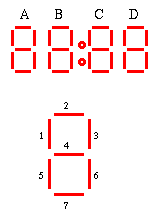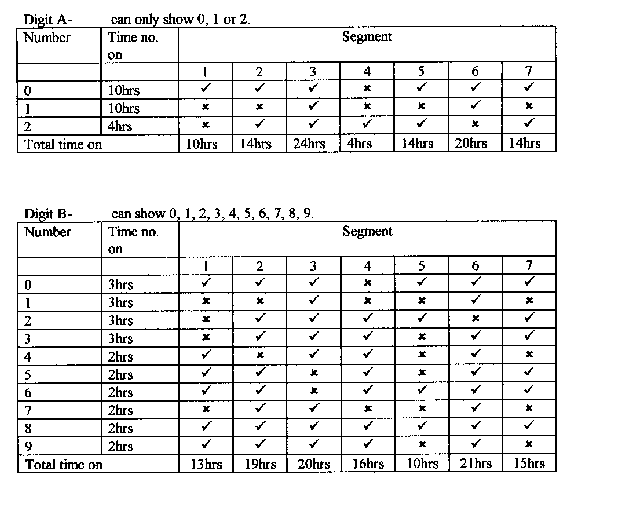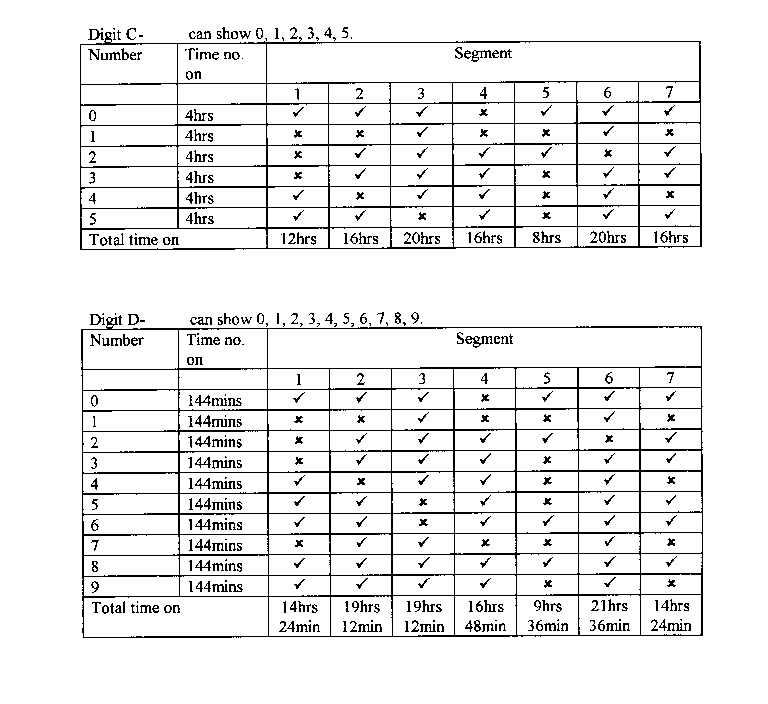#### You may also like### Speedy Sidney

Two trains set off at the same time from each end of a single straight railway line. A very fast bee starts off in front of the first train and flies continuously back and forth between the two trains. How far does Sidney fly before he is squashed between the two trains?### Rule of Three

If it takes four men one day to build a wall, how long does it take 60,000 men to build a similar wall?### Crossing the Atlantic

Every day at noon a boat leaves Le Havre for New York while another boat leaves New York for Le Havre. The ocean crossing takes seven days. How many boats will each boat cross during their journey?

# Digital Times

##### Age 11 to 14Challenge Level

Hayley of Lytham St Annes High School sent in a beautifully presented word processed solution which we have re-produced here. She did more than the question asked for, calculating how long each segment is lit up in a day rather than simply saying which is lit up for the most time and which for the least time. In the best mathematical style Hayley first set up her notation. Another very good solution was sent in by Helen of Maidstone Girls' Grammar School who used the same notation.In doing mathematics in real life (as opposed to answering text book or exam questions) the first step is to define the problem precisely and in so doing you must state clearly what is being assumed. This is exactly what Hayley did, she assumed that, until ten o'clock, rather than having no number lit up for digit A, a zero is put there. If you make different assumptions you get different versions of the problem which will, in many cases, have different answers.

• Solution to version 1 of the problem:
If you do not make Hayley's assumption then the question becomes much easier. In this version of the problem digit A never shows a zero. When A is lit up it shows a 1 for the 12 hour clock or a 1 or 2 for a 24 hour clock. In this case no segment is lit up all the time and segment 6 in digit D (the units digit for minutes) is lit for the most time for both 12 and 24 hour clocks (actually for nine tenths of the time). The segments lit up for least time on the 12 hour clock are segments 1,2,4,5 and 7 in digit A (the tens digit for hours) which are not lit at all, and for the 24 hour clock segment 1 in digit A which is still not used at all.
• Hayley's solution to version 2 of the problem.
In this version of the problem digit A always shows either 0, 1 or 2.
##### The 12 hour clock
Most Used Segment

Segments 3 and 6 on digit A are on 24 hours a day.
There is either a 0 or a 1 for digit A, and these two segments are lit for both a 0 and a 1.

It is easy to discount all other digits as they all cycle round every digit, or digits 1-5, and every segment is turned off at some time in this way.

Least Used Segment

Segment 4 on digit A is never turned on, as it is not contained in either the 0 or 1.

You can discount the other segments as they are all turned on at some point.

##### The 24 Hour Clock

From the following tables you can tell that the segment on for the most time is segment 3 on digit A. As this digit can only have a 0, 1, or 2, this segment is on all the time.

The least used segment on the 24 hour clock is segment 5 on digit C.